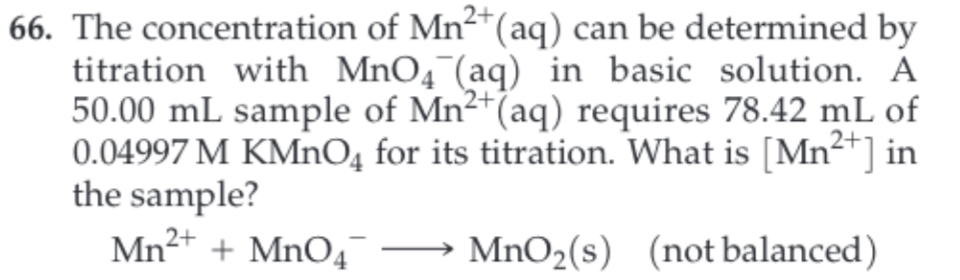# 66. The concentration of Mn2(aq) can be determined bytitration with MnO4 (aq) in basic solution. A50.00 mL sample of Mn2 (aq) requires 78.42 mL of0.04997 M KMnO4 for its titration. What is [Mn2 ] inthe sample?Mn2 MnO4MnO2(s) (not balanced)

Question
105 viewshelp_outlineImage Transcriptionclose66. The concentration of Mn2(aq) can be determined by titration with MnO4 (aq) in basic solution. A 50.00 mL sample of Mn2 (aq) requires 78.42 mL of 0.04997 M KMnO4 for its titration. What is [Mn2 ] in the sample? Mn2 MnO4 MnO2(s) (not balanced) fullscreen
check_circle

Step 1

The steps involved in the balancing of a redox equation are:

1. First, balance the number of electrons present on both the sides of the half-cell equation.
2. Add the appropriate number of water molecules to the opposite side of the chemical equation in order to balance the number of oxygen atoms.
3. Add the appropriate number of hydrogen ions to the opposite side of the chemical equation in order to balance the number of hydrogen atoms.
4. Add the balanced half-cell reactions in order to obtain a complete redox reaction.
5. The last step is to balance the charges on both sides of the half-cell reaction.

The molarity of an unknown solution can be determined by titrating it with a known solution by using the equation (1), in which, M1 is the molarity of the unknown solution, V1 is the volume of the unknown solution, M2 is the molarity of the known solution and V2 is the volume of the known solution.

Step 2

The given half-cell reactions are,

1. I) MnO4- → MnO2
2. II) Mn2+ → MnO2

Balance the (I) reaction as follows:

The oxidation state of manganese is changed from +7 to +4 on going from the reactant to the product side. Therefore, the number of electrons will be balanced on both sides by adding 3 electrons to the reactant side.

MnO4- + 3e- → MnO2

Add 2 water molecules on the product side in order to balance the number of oxygen atoms.

MnO4- + 3e- → MnO2 + 2H2O

Add 4 hydrogen ions on the reactant side in order to balance the number of hydrogen atoms.

MnO4- + 4H+ + 3e- → MnO2 + 2H2O              …… (III)

Step 3

Balance the (II) reaction as follows:

The oxidation state of manganese is changed from +2 to +4 on going from the reactant to the product side. Therefore, the number of electrons will be balanced on both sides by adding 2 electrons to the product side.

Mn2+ → MnO2 + 2e-

Add 2 water molecules on the reactant side in order to balance the number of oxygen atoms.

Mn2+ + 2H2O →...

### Want to see the full answer?

See Solution

#### Want to see this answer and more?

Solutions are written by subject experts who are available 24/7. Questions are typically answered within 1 hour.*

See Solution
*Response times may vary by subject and question.
Tagged in

### Chemistry